﻿ Resonance of CantileverExamples | Product | Murata Software Co., Ltd.Example14Resonance of Cantilever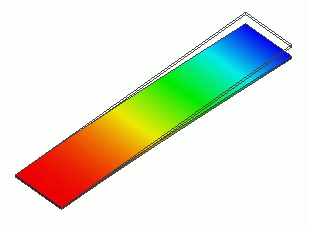General

• The resonance of a cantilever is analyzed. Unlike Exercises 11 through 13,
one end is fixed with the displacement boundary condition.

• The deformation, the displacement and the mechanical stress are solved.

• The directional sensitivity due to the participation factor and the effective mass can be examined for obtained modes.

• Unless specified in the list below, the default conditions will be applied.

Analysis Space

 Item Settings Analysis Space 3D Model unit mm

Analysis Conditions

The analysis type is the harmonic analysis.

 Item Settings Solvers Mechanical Stress Analysis [Galileo] Analysis Type Resonant analysis Options N/A

The resonant analysis tab is set up as follows.

 Tab Setting Item Settings Resonant analysis Number of modes 3 Approximated frequency 0[Hz]

Model

The cantilever is created as a box-shape solid body.

The material is silicon. One end is fixed with the displacement boundary condition.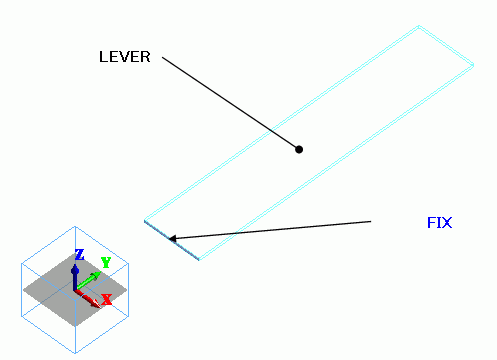Body Attributes and Materials

 Body Number/Type Body Attribute Name Material Name 0/Solid LEVER 301_Silicon(single-crystal) *

* Available from the Material DB

Boundary Conditions

 Boundary Condition Name/Topology Tab Boundary Condition Type Settings FIX/Face Mechanical Displacement Select all X/Y/Z components. UX=0, UY=0, UZ=0

Results

The following will be output on the output window or the log file.

 <> Eigenvalue (resonant frequency):[Hz] Mode= 2.50953498e+004 Mode= 1.65669006e+005 Mode= 3.22935422e+005

The resonant frequencies can be checked on Table.

The displacement diagram of Mode is shown below. The contour is the Z displacement.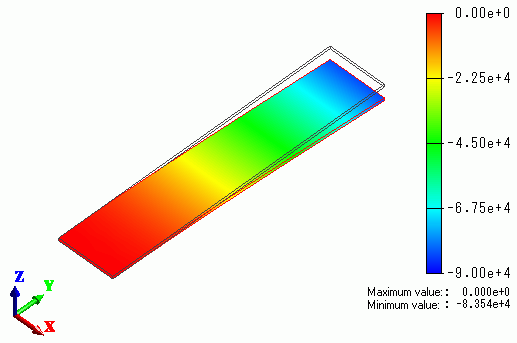The displacement diagram of Mode is shown below. The contour is the Z displacement.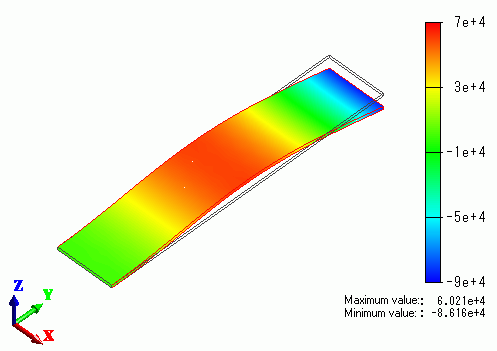The effective mass ratio graph can be output from [Table].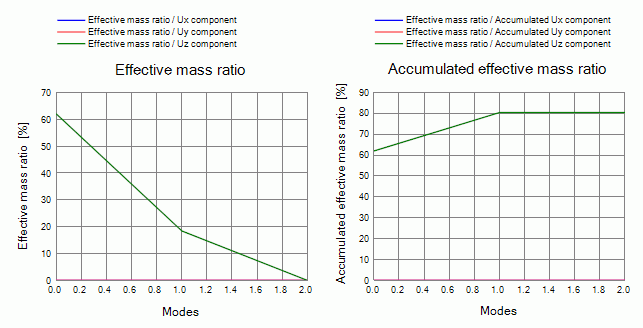UZ component (the effective mass ratio in Z direction) is 62.0[%] at Mode 0, 18.4[%] at Mode 1 and 0[%] at Mode 2.

Mode 0 is the main mode.

The accumulated value is 80.4[%].

If more modes are calculated, it will approach 100[%].

As for UX and UY components, the main modes are not obtained yet.

You need to calculate more modes.

﻿